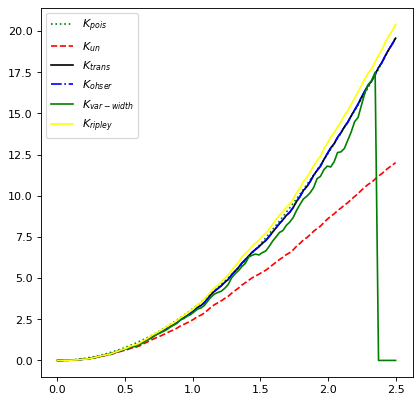# Ripley’s K Function Estimators¶

Spatial correlation functions have been used in the astronomical context to estimate the probability of finding an object, e.g. a galaxy, within a given distance of another object .

Ripley’s K function is a type of estimator used to characterize the correlation of such spatial point processes , , , , . More precisely, it describes correlation among objects in a given field. The RipleysKEstimator class implements some estimators for this function which provides several methods for edge-effects correction.

## Basic Usage¶

The actual implementation of Ripley’s K function estimators lie in the method evaluate which take the following arguments data, radii, and, optionally, mode.

The data argument is a 2D array which represents the set of observed points (events) in the area of study. The radii argument corresponds to a set of distances for which the estimator will be evaluated. The mode argument takes a value on the following linguistic set {none, translation, ohser, var-width, ripley}; each keyword represents a different method to perform correction due to edge-effects. See the API documentation and references for details about these methods.

Instances of RipleysKEstimator can also be used as callables (which is equivalent to calling the evaluate method).

A minimal usage example is shown as follows:

import numpy as np
from matplotlib import pyplot as plt
from astropy.stats import RipleysKEstimator

z = np.random.uniform(low=5, high=10, size=(100, 2))
Kest = RipleysKEstimator(area=25, x_max=10, y_max=10, x_min=5, y_min=5)

r = np.linspace(0, 2.5, 100)
plt.plot(r, Kest.poisson(r), color='green', ls=':', label=r'$K_{pois}$')
plt.plot(r, Kest(data=z, radii=r, mode='none'), color='red', ls='--',
label=r'$K_{un}$')
plt.plot(r, Kest(data=z, radii=r, mode='translation'), color='black',
label=r'$K_{trans}$')
plt.plot(r, Kest(data=z, radii=r, mode='ohser'), color='blue', ls='-.',
label=r'$K_{ohser}$')
plt.plot(r, Kest(data=z, radii=r, mode='var-width'), color='green',
label=r'$K_{var-width}$')
plt.plot(r, Kest(data=z, radii=r, mode='ripley'), color='yellow',
label=r'$K_{ripley}$')


(png, svg, pdf)